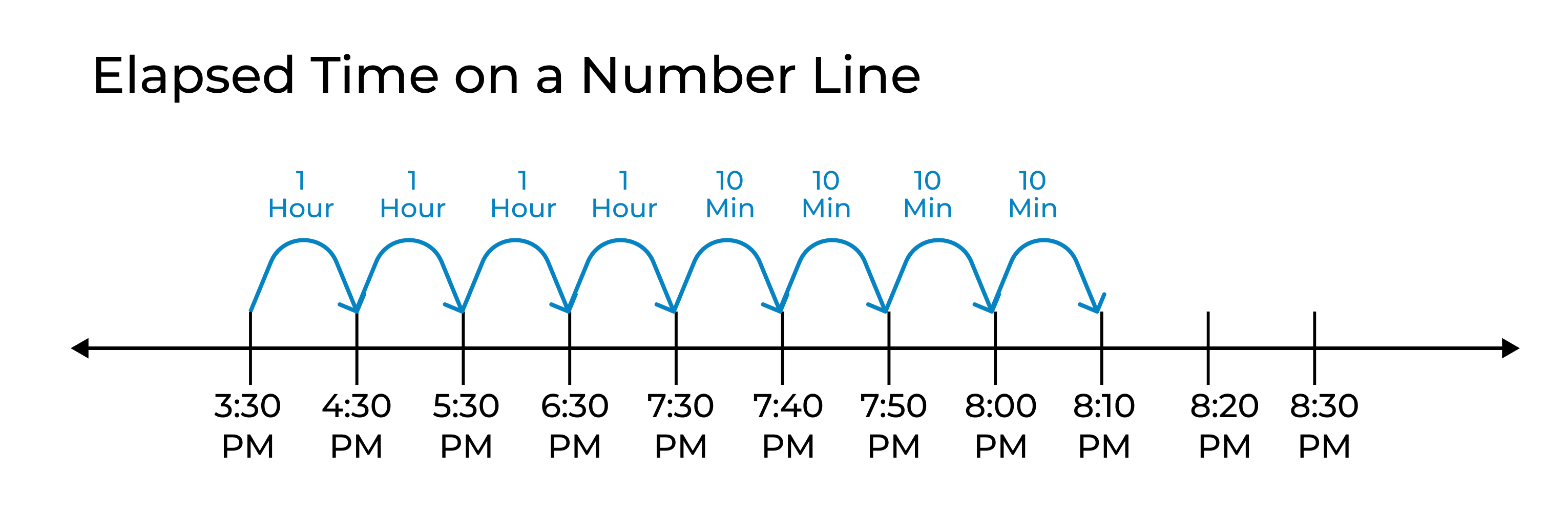Uh Oh! It seems you’re using an Ad blocker!

We always struggled to serve you with the best online calculations, thus, there's a humble request to either disable the AD blocker or go with premium plans to use the AD-Free version for calculators.

Or# Elapsed Time Calculator

Find out the elapsed time by just providing the start time and end time to this calculator.

Elapsed Time
Clock Time

Start Time:

Sec:

hrs:

mins:

sec:

End Time:

Sec:

hrs:

mins:

sec:

Table of Content

Get the Widget!

Add this calculator to your site and lets users to perform easy calculations.

Feedback

How easy was it to use our calculator? Did you face any problem, tell us!

Use the elapsed time calculator to determine how many hours, minutes, and seconds are there between the start and end time of an event.

With the help of this handy tool, you can determine the elapsed time between two clock readings in a matter of seconds.

## What Is An Elapsed Time?

“This is the time that passes from the start of an event to its end”

Mostly, it is used to measure the duration of an event or process. Elapsed time is widely used in various fields such as sports, science, transportation, and especially in managing projects.

Elapsed time is measured in hours, minutes, and seconds and you can calculate it manually with the help of the formula or can calculate it conveniently with the help of a time elapsed calculator.

### Elapsed Time Formula:

The elapsed time equation is as follows:

ET = ET_1 − ET_0

Where

• ET represents the elapsed time
• ET_1 is the end time of an event
• ET_0 is the representative of the start time

## Why Is It Important To Know Elapsed Time?

It’s an important concept that helps in:

• Learning to read the time from the clock
• Help us plan when we should leave to reach a specific place
• This allows us to schedule the time efficiently

To perform the calculations quickly, get the help of a time duration calculator hours. You will just have to provide a few simple inputs and it will perform the rest calculation for you.

## How To Calculate Elapsed Time?

Go through the following steps to calculate the elapsed time manually:

• First of all, determine the start time and end time of the event. Keep in mind it’s necessary to use the same time format such as 24 hours or 12 hours.
• If the start and end times are not in the same format then convert one format to the other to bring both times in the same format.
• Put the values of times in the above-mentioned elapsed time formula and subtract the start time from the end time as we have done in the following example.

### Example:

Suppose an event starts at 6:00 AM and ends at 11:00 AM, find the time elapsed of the event.

#### Solution:

Given that:

Start Time = 06:00 AM

End Time = 11:00 AM

Both times are in the same format, put these values in the elapsed time formula:

ET = ET_1−ET_0

ET = 11:00 – 06:00 = 05:00

ET = 5 hours

Rather than dragging yourself into this manual calculation, get the help of a time elapsed calculator. It will let you find out the time duration between two times without much manual intervention.

## How Does Our Elapsed Time Calculator Work?

This time in between calculator will let you calculate the time duration between two times by just providing a few inputs. Let’s see how it works!

### What Do You Need to Enter?

• Start Time: The time at which a specific event or process starts in hh:mm:ss
• End Time: The time at which the event ends up in hh:mm:ss

### This Is What You Will Get!

• Duration
• Elapsed time
• Duration in different units

This time passed calculator is solely designed to calculate the elapsed time so that you can solve all of your time-elapsed word problems.

Don’t waste your time in manual calculations, just access this hour duration calculator from your browser and determine how much time is between two times.

## FAQ’s:

### How To Calculate Elapsed Time on A Number Line?

Calculate elapsed time on a number line by marking 1-hour intervals and when you reach the last hour, then divide it into 10-minute intervals.### How To Solve Word Problems of Elapsed Time?

Solving word problems of time elapsed includes finding the time that has passed between the start and end time. You can do that by yourself or with the help of an elapsed time calculator. To solve them follow the below-mentioned tips:

• Determine the start and end times of the event
• Convert times to the same format (if needed)
• Calculate the elapsed time

### Is Elapsed Time Same As Duration?

Yes, but according to the elapsed time definition, it Is used in the context of calculating the time a task, activity, or event has taken to complete, and on the other hand, the duration refers to the time frame of an event.

### What Is Estimated Elapsed Time?

This is the estimated completion time of an event or process. Mostly, it is used for estimating the duration of flights.

## References:

cuemath.com: Elapsed Time.

byjus.com: What is Elapsed Time?